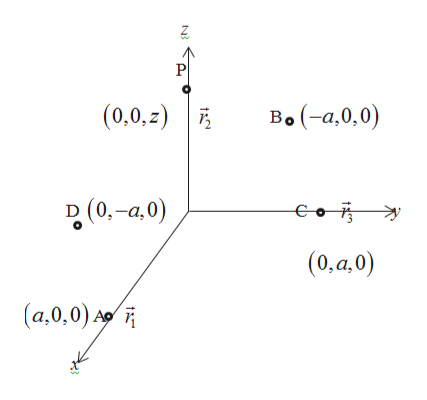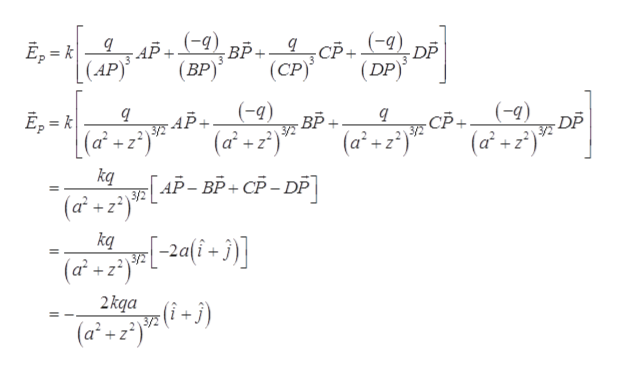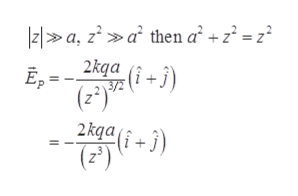3-a) Consider the following configuration of point charges: charge q at ~r1 = (a, 0, 0); charge −qat ~r2 = (−a, 0, 0); charge q at ~r3 = (0, a, 0); and finally charge −q at ~r4 = (0, −a, 0); so a total offour point charges. Determine the electric field E~ at a generic point on the z-axis. Discuss whathappens when |z|  a.b) Consider the following configuration of point charges: Charge q at ~r1 = (0, 0, a); charge q at~r2 = (0, 0, −a); and finally charge −2q at ~r3 = (0, 0, 0); so a total of three point charges. Determinethe electric field E~i) at a generic point on the x-axis. Discuss what happens when |x|  a,ii) at a generic point on the z-axis. Discuss what happens when |z|  a.

Question

3-a) Consider the following configuration of point charges: charge q at ~r1 = (a, 0, 0); charge −q
at ~r2 = (−a, 0, 0); charge q at ~r3 = (0, a, 0); and finally charge −q at ~r4 = (0, −a, 0); so a total of
four point charges. Determine the electric field E~ at a generic point on the z-axis. Discuss what
happens when |z|  a.
b) Consider the following configuration of point charges: Charge q at ~r1 = (0, 0, a); charge q at
~r2 = (0, 0, −a); and finally charge −2q at ~r3 = (0, 0, 0); so a total of three point charges. Determine
the electric field E~
i) at a generic point on the x-axis. Discuss what happens when |x|  a,
ii) at a generic point on the z-axis. Discuss what happens when |z|  a.

Step 1

(a)

The figure 1 shows the generic point charges.help_outlineImage TranscriptioncloseP Bo (-а,0,0) (0,0,z) B(0,-a,0) -Goi (0,а,0) (a,0,0) t fullscreen
Step 2

The electric field at a generic point on the z axis is,

Substitute the point values from the given figure.help_outlineImage TranscriptioncloseЕ, - к (АP) АР+ 9) вр. 9 СР+ 9) DP (DP) (ВР)" (СР) (-9) ВР Е, - к (a2z° (-9) У -АР- (af + Г АР-ВР-СР-DP] (Б- DP -СР. /2 УЕ (a°2) 3/Е +z (af+) kq 3/2 (a2 z + kq (а' +2)" 2kqa (i -) 3/72 (a2+z2) fullscreen
Step 3

According to the given condition,...help_outlineImage Transcriptionclose|z1a, za then a +z = z° 2kga (i j) E,= (2) 2 kqai+3 (2) = fullscreen

Want to see the full answer?

See Solution

Want to see this answer and more?

Our solutions are written by experts, many with advanced degrees, and available 24/7

See Solution
Tagged in

Electric Charges and Fields